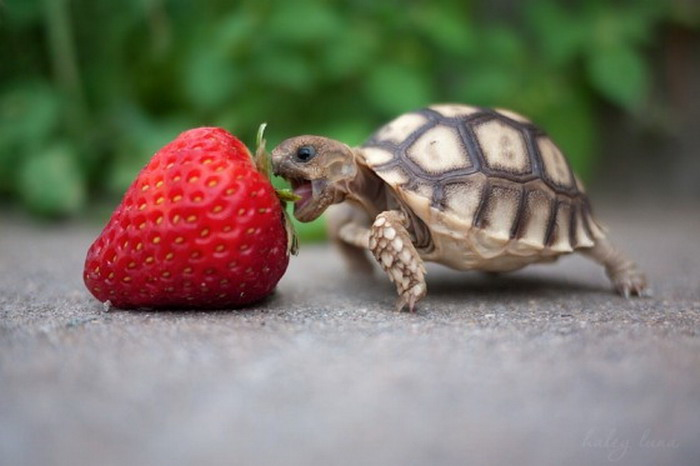Play

### Dr. Manthey's profoundly silly contribution.

The cat jumped in the hat, $x+2$.

(1)
\begin{align} \int_0^1 x^2 dx = \frac{1}{3} \end{align}### Lisa

Hello from Lisa! $x^2+2x+3$ :)

### Alexei

(2)
\begin{align} \int_1^2 x^3+2x^2+3x+4 dx = \frac{203}{12} \end{align}

Hi from Megan! $x^3+4x^2+3x+5$
I thought I would try to insert a picture. Here is a little kitty for everyone to enjoy!Hi from Amanda!
Here's my mathematical equation $a^2+b^2=c^2$

### Brianna

Bri is trying this out now! Barewith me…
$f(x)=x^5+y$

Hello from Vivian!! My Math equation is: $x^4+x^3+x^2+4$### Jessica

Hellllllo fellow math nerds, excuse me, mathletes! $5x^5+4x^4+3x^3+2x^2 +x$